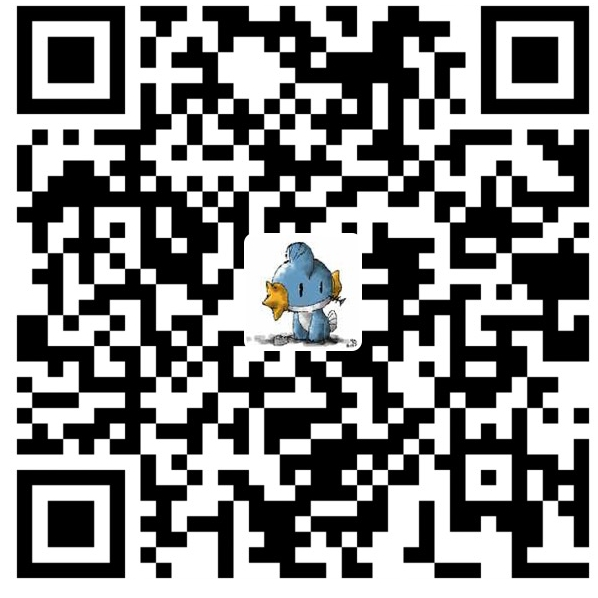# VBS基础教程第二篇

const PI=3.1415926

const NAME="记忆碎片"

msgbox("Hello World")
msgbox("Hello World")
msgbox("Hello World")
msgbox("Hello World")
msgbox("Hello World")

const hw="Hello World"
msgbox(hw)
msgbox(hw)
msgbox(hw)
msgbox(hw)
msgbox(hw)

Dim a1,a2,a3
a1=14
a2=12.23
a3="Hello"

int a1; Dim a1 as integer
float a2; Dim a2 as Double
char* a3; Dim a3 as strnig
a1=14; a1=14
a2=12.23; a2=12.23
a3="Hello"; a3="Hello"

dim a,b,s
a=15
b=12
s=a*b
msgbox(s)

dim s
s=15*12
msgbox(s)

dim a,b,s
a=inputbox("请输入矩形的长:")
b=inputbox("请输入矩形的宽:")
s=a*b
msgbox(s)

ok, 这么一修改, 无论用户输入怎样的数据, 我们都能计算出矩形的面积了. 如果你用s=15*12能改嘛? 当然不行.

dim ans
ans=12+32/4+[(23-10)*2]
msgbox(ans)

dim a
a=16 mod 5

dim a,b,c
a=2
b=a^2
c=a^3
msgbox(a)
msgbox(c)

1) 常量分为自然常量和自定义常量, 常量的值不可修改

2) 变量就像盒子, 我们不在乎盒子里装的是什么, 但一定要知道盒子的名字

3) 四则运算在编程中没有任何不同

4) MOD是取余运算

1) 编一个程序, 计算圆形的面积, 半径由用户给出 (使用Inputbox) PI取值3.14159

2) 编一个程序取得20 / 3 的余数微信赞赏支付宝赞赏

### 一条评论 发表在“VBS基础教程第二篇”上

1.Icy_Ybk说道：

“我想你已经发现了, vbs中的数学计算和真正的算术没有什么不同, +,-,*,/,(),[],{}都是一样的用法, 比如:

dim ans
ans=12+32/4+[(23-10)*2]
msgbox(ans)”

Demon 大神，这句似乎有些问题吧，方框中的内容在这里被视为一个变量名了，返回的是 0， ans 计算得到 20。实际上应该得到 46。VBS 的括号嵌套仅能对小括号进行嵌套。而 {} 这样的括号在 VBS 里是不能用的。
相信 Demon 大神应该早就知道这一点啊。。。嗯，估计是当时一不小心写错了。